# deepgp: an R-package for Bayesian Deep Gaussian Processes

library(deepgp)

# Overview

The deepgp package provides a fully-Bayesian implementation of deep Gaussian process (DGP) regression models via Markov Chain Monte Carlo (MCMC). The package was designed with an eye towards surrogate modeling of computer experiments. It supports several acquisition criteria for strategic sequential design and offers optional Vecchia-approximation for faster computations with larger data sizes. Computations are performed in C/C++ under-the-hood, with optional parallelization through OpenMP and SNOW.

This vignette serves as an introduction to the package's main features. Full methodological details are provided in the following manuscripts:

• Sauer, Gramacy, and Higdon (2022) : Bayesian DGPs with sequential design targeting minimized posterior variance
• Sauer, Cooper, and Gramacy (2022) : incorporation of the Vecchia approximation into the Bayesian DGP framework
• Gramacy, Sauer, and Wycoff (2021) : optimization through expected improvement with strategic selection of candidates

While I will showcase simple one-dimensional examples here, the simulated examples provided in these works span up to six dimensions, with training data sizes up to tens of thousands. Real-world applications to computer experiments include a three-dimensional simulation of the Langley Glide Back Booster (LGBB, Pamadi et al. 2004) and the seven-dimensional Test Particle Monte Carlo Simulator (TPM) of a satellite in low earth orbit (Sun et al. 2019), with training data sizes ranging from $$n = 300$$ to $$n = 100,000$$.

All exercises are supported by code in a public git repository: https://bitbucket.org/gramacylab/deepgp-ex/. Further details on function arguments and default settings (including prior distributions) are provided in the function documentation.

In the first section, I'll discuss model fitting (training through MCMC then generating posterior predictions). I'll review a typical one-layer Gaussian process before introducing the DGP functionality. I'll also discuss extensions to the original DGP framework, including the utilization of Vecchia approximation (Vecchia 1988) for faster computations. In the second section, I'll introduce sequential design criteria for two different objectives: optimizing the response of minimizing posterior variance.

To start, let's define a one-dimensional function that we can use for demonstration and visualization. The following code defines the "Higdon function" (D. Higdon 2002), found on the pages of the Virtual Library of Simulation Experiments (Surjanovic and Bingham 2013).

higdon <- function(x) {
i <- which(x <= 0.6)
x[i] <- 2 * sin(pi * 0.8 * x[i] * 4) + 0.4 * cos(pi * 0.8 * x[i] * 16)
x[-i] <- 2 * x[-i] - 1
return(x)
}

Next, we generate training and testing data. For now, we will work in a noise-free setting (although we will presume not to know that there is no noise).

# Training data
n <- 24
x <- seq(0, 1, length = n)
y <- higdon(x)

# Testing data
np <- 100
xp <- seq(0, 1, length = np)
yp <- higdon(xp)

plot(xp, yp, type = "l", col = 4, xlab = "X", ylab = "Y", main = "Higdon function")
points(x, y)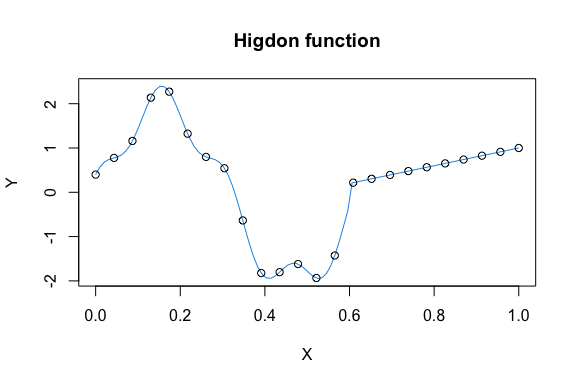The piecewise form of this function is an example of "non-stationarity". There are two distinct regimes (one wiggly and one linear), with an abrupt shift at x = 0.6. We will soon see how a one-layer GP suffers from an assumption of stationarity which restricts it from fitting these two separate regimes simultaneously. DGPs are better equipped to handle such complex surfaces.

# Model Fitting

## One-layer Shallow GP

Traditional Gaussian processes (GPs) are popular nonlinear regression models, preferred for their closed-form posterior predictive moments, with relevant applications to Machine Learning (ML, Rasmussen and Williams 2005) and computer experiment surrogate modeling (Santner, Williams, and Notz 2018; R. B. Gramacy 2020). GP models place a multivariate normal prior distribution for the response, $Y \sim \mathcal{N}\left(0, \Sigma(X)\right),$ with $\Sigma(X)^{ij} = \Sigma(x_i, x_j) = \tau^2\left(k\left(\frac{||x_i - x_j||^2}{\theta}\right) + g\mathbb{I}_{i=j}\right),$ where $$k(\cdot)$$ represents a stationary kernel function (deepgp offers both the squared exponential and Matérn kernels). The package only supports mean-zero priors, but non-zero means may be addressed by pre-scaling the response appropriately. In this canonical form, GPs suffer from the limitation of stationarity due to reliance on covariance kernels $$k(\cdot)$$ that are strictly functions of Euclidean distance.

This covariance depends on hyperparameters $$\tau^2$$, $$\theta$$, and $$g$$ controlling the scale, lengthscale, and noise respectively. [Notice how $$\theta$$ operates on squared distances under this parameterization.] Looking forward to our DGP implementation, we embrace an MCMC scheme utilizing Metropolis-Hastings sampling of these unknown hyperparameters (except $$\tau^2$$ which can be marginialized analytically under an inverse gamma reference prior, see R. B. Gramacy (2020), Chapter 5).

Ok, let's get to some code. MCMC sampling of theta and g for a one-layer GP is built into the fit_one_layer function. The following code collects 10,000 Metropolis Hastings samples (using the Matérn kernel by default and creating an S3 object of the gp class), then displays trace plots of the samples.

fit1 <- fit_one_layer(x, y, nmcmc = 10000, verb = FALSE)
plot(fit1)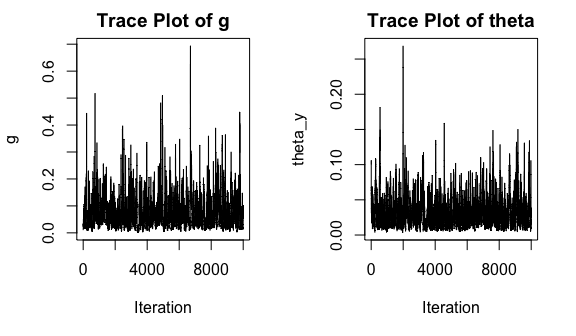There is randomization involved in MCMC proposals and acceptances, so these trace plots may show slight variations upon different renderings. In this simple case they burn-in quickly. Before we do anything else with these samples, we may want to trim off burn-in and thin the remaining samples. This functionality is built into the trim function (which will work on any of our models, not just the one layer).

fit1 <- trim(fit1, 5000, 2) # remove 5000 as burn-in, thin by half

Now that we have a set of posterior samples for theta and g, we can generate posterior predictions for the testing locations xp. Under a GP prior, posterior predictions $$Y^\star$$ at $$X^\star$$ locations follow \begin{aligned} Y^\star \mid X, Y \sim \mathcal{N}\left(\mu^\star, \Sigma^\star\right) \quad\textrm{where}\quad \mu^\star &= \Sigma(X^\star, X)\Sigma(X)^{-1}Y \\ \Sigma^\star &= \Sigma(X^\star) - \Sigma(X^\star, X)\Sigma(X)^{-1}\Sigma(X, X^\star) \end{aligned}

Each $$\Sigma(\cdot)$$ above relies on values of $$\theta$$ and $$g$$. Since we have MCMC samples $$\{\theta^t, g^t\}$$ for $$t\in\mathcal{T}$$, we can evaluate $$\mu^{\star(t)}$$ and $$\Sigma^{\star(t)}$$ for each $$t\in\mathcal{T}$$ and aggregate the results using the law of total variance, $\mu^\star = \sum_{t=1}^\mathcal{T} \mu^{\star(t)} \quad\textrm{and}\quad \Sigma^\star = \sum_{t=1}^\mathcal{T} \Sigma^{\star(t)} + \mathbb{C}\mathrm{ov}\left(\mu^{\star(t)}\right)$

This process is neatly wrapped in the predict function (tailored for each S3 class object), with convenient plotting in one-dimension provided in the plot function.

fit1 <- predict(fit1, xp, lite = FALSE)
plot(fit1)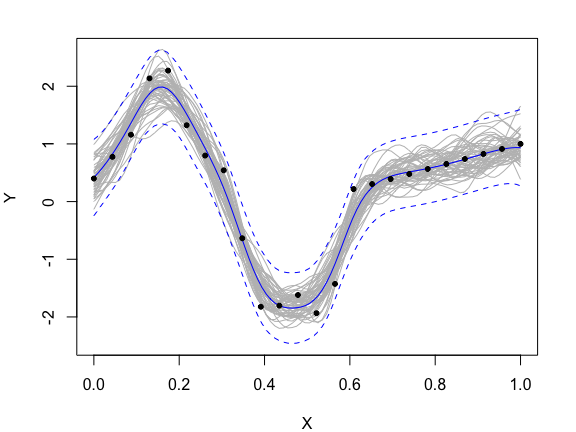The GP provides a nice nonlinear fit with appropriate uncertainty estimates, but it fails to pick up on the regime shifts. We allowed the model to estimate a noise parameter, and it over-smoothed the data.

## Two-layer DGP

DGPs address this stationarity limitation through functional compositions of Gaussian layers. Intermediate layers act as warped versions of the original inputs, allowing for non-stationary flexibility. A "two-layer" DGP may be formulated as \begin{aligned} Y \mid W &\sim \mathcal{N}\left(0, \Sigma(W)\right) \\ W_i &\sim \mathcal{N}\left(0, \Sigma_i(X)\right) \quad\textrm{for}\quad i = 1, \dots, D \end{aligned} where $$\Sigma(\cdot)$$ represents a stationary kernel function as defined in the previous subsection and where $$W = [W_1, W_2, \dots, W_D]$$ is the column-combined matrix of individual $$W_i$$ "nodes". Latent layers are noise-free with unit scale (i.e. $$g = 0$$ and $$\tau^2 = 1$$ for each $$W_i$$). The intermediate latent layer $$W$$ is unobserved and presents an inferential challenge (it can not be marginialized from the posterior analytically). Many have embraced variational inference for fast, thrifty approximations (Salimbeni and Deisenroth 2017,Marmin and Filippone (2022)). The deepgp package offers an alternative, fully-Bayesian sampling-based inferential scheme as detailed in Sauer, Gramacy, and Higdon (2022). This sampling approach provides full uncertainty quantification (UQ), which is crucial for sequential design.

Latent Gaussian layers are sampled through elliptical slice sampling (ESS, Murray, Adams, and MacKay 2010). Kernel hyperparameters are sampled through Metropolis Hastings. All of these are iterated in a Gibbs scheme. MCMC sampling for two-layer DGPs is wrapped in the fit_two_layer function.

fit2 <- fit_two_layer(x, y, nmcmc = 8000, verb = FALSE)
plot(fit2)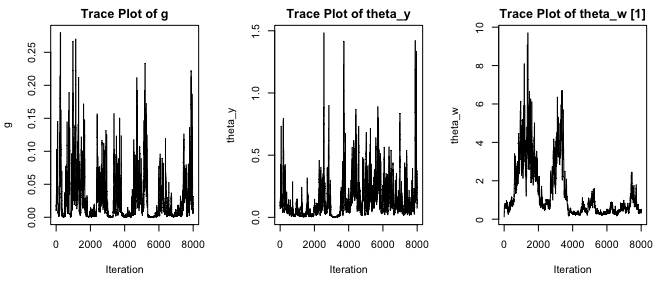Notice there are now two lengthscale parameters, one for each GP layer (there will be additional theta_w[i] in higher dimensions, with each $$\Sigma_i(X)$$ having its own). Again there is some randomization involved in this sampling. These trace plots are helpful in diagnosing burn-in. If you need to run further MCMC samples, you may use the continue function.

fit2 <- continue(fit2, 2000, verb = FALSE)

There are now 10,000 total samples stored in fit2,

fit2nmcmc #>  10000 In one dimension we may visualise the ESS samples of W by specifying hidden = TRUE to the plot call. plot(fit2, trace = FALSE, hidden = TRUE)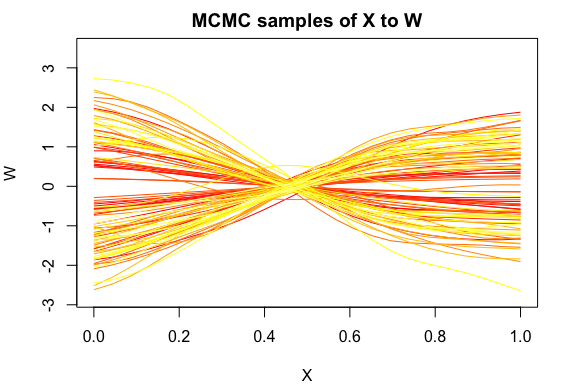Each line represents a single ESS sample of $$W$$. Since 10,000 lines would be a lot to plot, an evenly spaced selection of samples is shown. The samples are colored on a gradient -- red lines are the earliest iterations and yellow lines are the latest. In this simple example these lines don't look all too interesting, but we see that they are "stretching" inputs in the left region (indicated by a steeper slope) and "squishing" inputs in the right region. This accommodates the fact that there is more signal for x < 0.6. We may then trim/thin and predict using the same function calls. Predictions follow the same structure of the one-layer GP, they just involve an additional mapping of $$X^\star$$ through $$W$$ before obtaining $$\mu^\star$$ and $$\Sigma^\star$$. We utilize lite = FALSE here so that full posterior predictive covariances are returned (by default, only point-wise variances are returned). fit2 <- trim(fit2, 5000, 2) fit2 <- predict(fit2, xp, lite = FALSE) plot(fit2)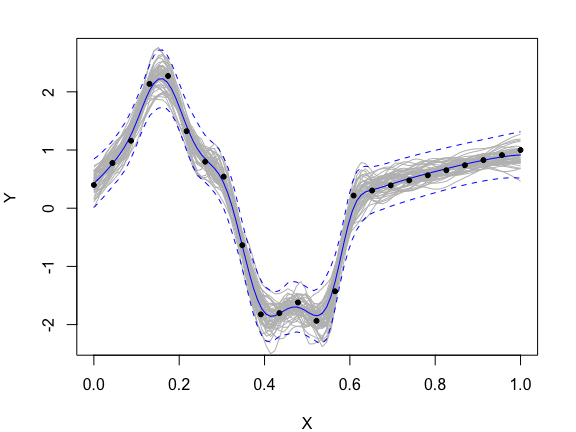The two-layer DGP was more easily able to identify the "wiggly" regions on the left, while simultaneously predicting the linear fit on the right. We allowed our DGP to also estimate the noise parameter, so it too over-smoothed a bit. ## Three-layer DGP A three-layer DGP involves an additional functional composition, \begin{aligned} Y \mid W &\sim \mathcal{N}\left(0, \Sigma(W)\right) \\ W_i \mid Z &\sim \mathcal{N}\left(0, \Sigma_i(Z)\right) \quad\textrm{for}\quad i = 1, \dots, D \\ Z_j &\sim \mathcal{N}\left(0, \Sigma_j(X)\right) \quad\textrm{for}\quad j = 1, \dots, D \end{aligned} We conduct additional Metropolis Hastings sampling of lengthscale parameters for the innermost layer and additional elliptical slice sampling for $$Z$$. In prediction, we incorporate an additional mapping $$X^\star \rightarrow Z \rightarrow W$$ before obtaining predictions for $$Y^\star$$. Training/prediction utilizes the same functionality, simply with the fit_three_layer function. fit3 <- fit_three_layer(x, y, nmcmc = 10000, verb = FALSE) fit3 <- trim(fit3, 5000, 2) fit3 <- predict(fit3, xp, lite = FALSE) plot(fit3)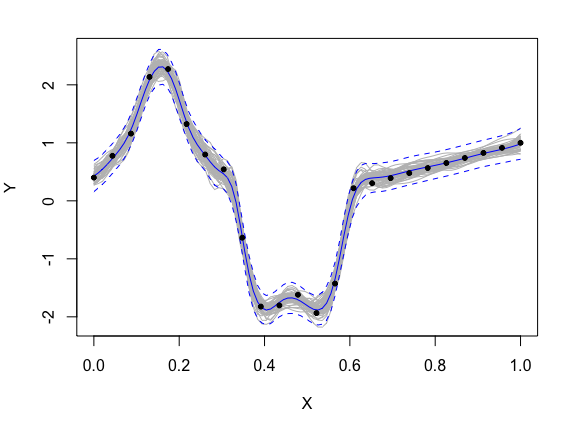You may notice that the three-layer fit is similar to the two-layer fit (although it requires significantly more computation). There are diminishing returns for additional depth. We will formally compare the predictions next. ## Evaluation Metrics To objectively compare various models, we can evaluate predictions against the true response at the testing locations. There are three evaluation metrics incorporated in the package: • rmse: root mean squared prediction error (lower is better) • crps: continuous rank probability score (requires point-wise predictive variances, lower is better) • score: predictive log likelihood (requires full predictive covariance, higher is better) Let's calculate these metrics for the four models we have entertained. metrics <- data.frame("RMSE" = c(rmse(yp, fit1mean),
rmse(yp, fit2$mean), rmse(yp, fit3$mean)),
"CRPS" = c(crps(yp, fit1$mean, diag(fit1$Sigma)),
crps(yp, fit2$mean, diag(fit2$Sigma)),
crps(yp, fit3$mean, diag(fit3$Sigma))),
"SCORE" = c(score(yp, fit1$mean, fit1$Sigma),
score(yp, fit2$mean, fit2$Sigma),
score(yp, fit3$mean, fit3$Sigma)))
rownames(metrics) <- c("One-layer GP", "Two-layer DGP", "Three-layer DGP")
metrics
#>                       RMSE       CRPS    SCORE
#> One-layer GP    0.19375467 0.12445641 2.023996
#> Two-layer DGP   0.08187180 0.06806456 2.980133
#> Three-layer DGP 0.06562534 0.04712727 3.762263

The three-layer outperforms the two-layer which in turn outperforms the one-layer across the board.

## Extensions

### Vecchia Approximation

The Bayesian DGP suffers from the computational bottlenecks that are endemic in typical GPs. Inverses of dense covariance matrices experience $$\mathcal{O}(n^3)$$ costs. The Vecchia approximation (Vecchia 1988) circumvents this bottleneck by inducing sparsity in the precision matrix (Katzfuss et al. 2020; Katzfuss and Guinness 2021). The sparse Cholesky decomposition of the precision matrix is easily populated in parallel (the package uses OpenMP for this), making for fast evaluation of Gaussian likelihoods and posterior predictive moments. In addition to the original, un-approximated implementation, the deepgp package offers the option to utilize Vecchia approximation in all under-the-hood calculations. Vecchia approximation is triggered by specifying vecchia = TRUE in any of the fit functions.

fit2_vec <- fit_two_layer(x, y, nmcmc = 10000, vecchia = TRUE, m = 25)

The m argument controls the fidelity of the approximation. Details are provided in Sauer, Cooper, and Gramacy (2022). While the original implementation was only suitable for data sizes in the hundreds, the Vecchia option allows for feasible computations with data sizes up to a hundred thousand.

Note, this Vecchia implementation is targeted towards no-noise or low-noise settings. It has not been adapted for high-noise, low-signal situations yet.

### Noise-free Modeling

If we know that our training data is noise-free, we can tell the fit functions to conduct noise-free modeling by fixing $$g = 0$$. In actuality, there are numerical issues that come from fixing the nugget to be too small (especially with a smoother kernel). To avoid any numerical issues, I recommend using a small value such as $$g = 1e-4$$ instead. This parameter is specified by the argument true_g to either fit_one_layer, fit_two_layer, or fit_three_layer.

fit2_no_g <- fit_two_layer(x, y, nmcmc = 10000, true_g = 1e-4)

If you use trim, predict, and plot on this fit, you will see that the predictions interpolate the points. [If you are experiencing numerical issues or the fit is not interpolating the points, simply increase the value of true_g. This is most likely to happen in the one-layer GP because of its smoother fit.]

### Separable Lengthscales for One-layer GP

To allow for non-stationarity along axis-alignment, it is common to use a vectorized lengthscale with separate $$\theta_h$$ for each dimension of $$X$$ (see R. B. Gramacy (2020), Chapter 5). $\Sigma(X)^{ij} = \Sigma(x_i, x_j) = \tau^2\left(k\left(\sum_{h=1}^d \left(\frac{(x_{ih} - x_{jh})^2}{\theta_h}\right)\right) + g\mathbb{I}_{i=j}\right),$ While this is not the primary functionality of the deepgp package, a one-layer GP with separable lengthscales (sampled through additional Metropolis Hastings) may be a handy benchmark. Separable (anisotropic) lengthscales are triggered by the argument sep = TRUE to fit_one_layer.

fit1_sep <- fit_one_layer(x, y, nmcmc = 10000, sep = TRUE)

Note, separable lengthscales are not an option for two- and three-layer models as the latent layers provide enough flexibility to mimic vectorized $$\theta$$. Latent layer nodes are modeled isotropically to preserve identifiability and parsimony.

# Active Learning

Active learning (also called "sequential design") is the process of sequentially selecting training locations using a statistical "surrogate" model to inform acquisitions. Acquisitions may target specific objectives including optimizing the response or minimizing posterior variance. The non-stationary flexibility of a DGP, combined with full UQ through Bayesian MCMC, is well-suited for sequential design tasks. Where a typical stationary GP may end up space-filling, DGPs are able to target areas of high signal/high interest (Sauer, Gramacy, and Higdon 2022).

## Optimization through Expected Improvement

If the sequential design objective is to minimize the response, the expected improvement criterion (EI, Jones, Schonlau, and Welch 1998) is offered by specifying the argument EI = TRUE within the predict function. For the Higdon function example this yields

fit2 <- predict(fit2, xp, EI = TRUE)
plot(xp, fit2$EI, type = "l", ylab = "EI") points(xp[which.max(fit2$EI)], max(fit2$EI), pch = 17, cex = 1.5, col = 4)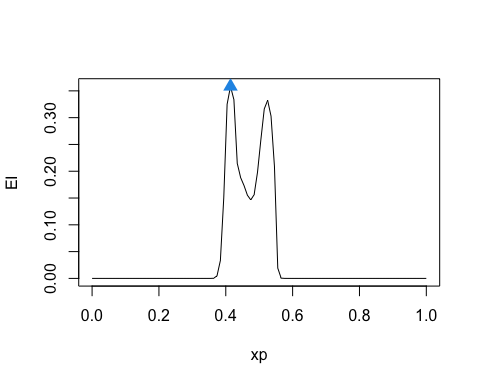where the next acquisition is chosen as the candidate that maximized EI (highlighted by the blue triangle). In this example, the EI criterion is accurately identifying the two local minimums in the response surface. This implementation relies on optimization through candidate evaluation. Strategically choosing candidates is crucial; see Gramacy, Sauer, and Wycoff (2021) for discussion on candidate optimization of EI with DGPs. ## Minimizing Posterior Variance If instead of optimizing, we simply want to obtain the best surrogate fit we may choose to conduct sequential design targeting minimal posterior variance. For the sake of keeping things interesting, let's introduce a new one-dimensional example to use for visualization in this section (and to demonstrate a noise-free situation). The following code defines a simple step function. f <- function(x) as.numeric(x > 0.5) # Training data x <- seq(0, 1, length = 8) y <- f(x) # Testing data xp <- seq(0, 1, length = 100) yp <- f(xp) plot(xp, yp, type = "l", col = 4, xlab = "X", ylab = "Y", main = "Step function") points(x, y)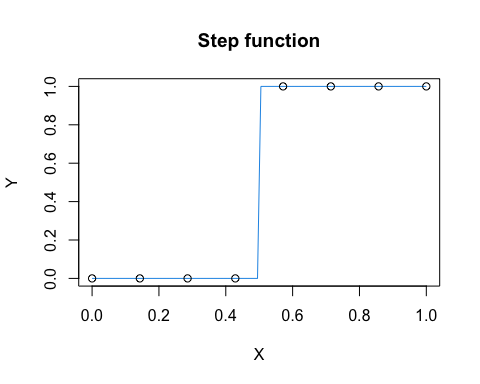We then fit one- and two-layer models, this time specifying the squared exponential kernel and fixing the noise parameter to a small value. fit1 <- fit_one_layer(x, y, nmcmc = 10000, cov = "exp2", true_g = 1e-4, verb = FALSE) fit1 <- trim(fit1, 5000, 5) fit1 <- predict(fit1, xp) plot(fit1)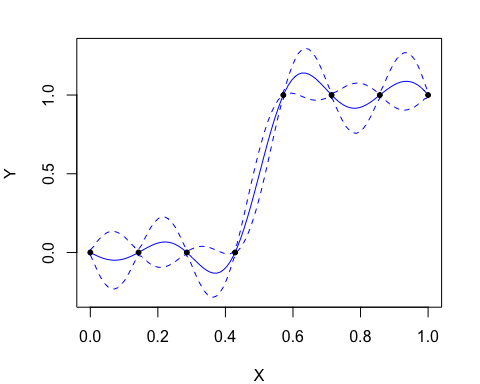fit2 <- fit_two_layer(x, y, nmcmc = 10000, cov = "exp2", true_g = 1e-4, verb = FALSE) fit2 <- trim(fit2, 5000, 5) fit2 <- predict(fit2, xp) plot(fit2)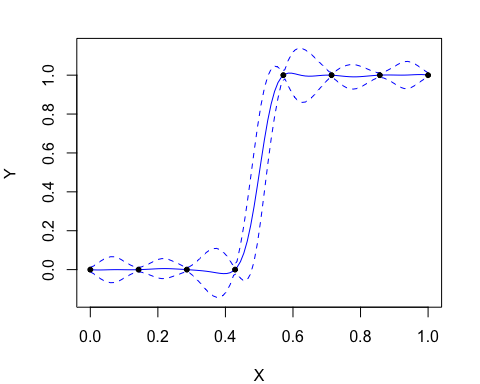Notice, the DGP model is better able to fit the stepwise nature of the response surface due to its nonstationarity flexibility. The deepgp package offers two acquisition criteria to target minimal posterior variance. The Integrated Mean Squared Error (IMSE) acquisition criterion (Sacks et al. 1989) is implemented in the IMSE function. The following code evaluates this criterion for the one- and two-layer models above using the predictive grid as candidates and plots the resulting values. The next acquisition is chosen as the candidate that produced the minimum IMSE (highlighted by the blue triangle). imse1 <- IMSE(fit1, xp) imse2 <- IMSE(fit2, xp) par(mfrow = c(1, 2)) plot(xp, imse1$value, type = "l", ylab = "IMSE", main = "One-layer")
points(xp[which.min(imse1$value)], min(imse1$value), pch = 17, cex = 1.5, col = 4)
plot(xp, imse2$value, type = "l", ylab = "IMSE", main = "Two-layer") points(xp[which.min(imse2$value)], min(imse2\$value), pch = 17, cex = 1.5, col = 4)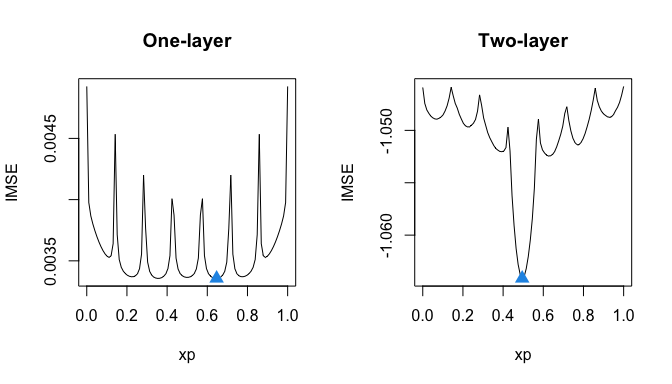Whereas the one-layer model is inclined to space-fill with low IMSE across most of the domain, the two-layer model appropriately targets acquisitions in the middle region, where uncertainty is highest. The sum approximation to IMSE, referred to as Active learning Cohn in the ML literature, is similarly implemented in the ALC function (although the ALC acquisition is chosen as the candidate that yields the maximum criterion value).

alc1 <- ALC(fit1, xp)
alc2 <- ALC(fit2, xp)

When conducting a full sequential design, it is helpful to initialize the DGP chain after an acquisition at the last sampled values of latent layers and kernel hyperparameters. Initial values of MCMC sampling for a two-layer DGP may be specified by the theta_y_0, theta_w_0, g_0, and w_0 arguments to fit_two_layer. Similar arguments are implemented for fit_one_layer and fit_three_layer. Examples of this are provided in the "active_learning" folder of the git repository (https://bitbucket.org/gramacylab/deepgp-ex/).

# Computational Considerations

Fully-Bayesian DGPs are hefty models that require a good bit of computation. While I have integrated tools to assist with fast computations, there are still some relevant considerations.

• If you are not using the Vecchia implementation, you may substantially speed-up under-the-hood matrix calculations by using a fast linear algebra library. R's default BLAS/LAPACK is not optimized for speedy matrix calculations. I recommend Intel's Math Kernel Library (MKL) when possible. Other options include OpenBLAS, the Accelerate framework on OSX, or Microsoft R Open. Check out Appendix A in R. B. Gramacy (2020) for further discussion and illustration of linear algebra library alternatives. Even with these, I recommend using vecchia = TRUE for data sizes above the low hundreds.
• If you are using the Vecchia implementation, computation speed is reliant on parallelizaton through OpenMP. When packages are installed from CRAN on a Mac, they are not compiled with OpenMP by default. To set up OpenMP parallelization on a Mac, download the package source code and install using the gcc/g++ compiler. The fit functions will produce a warning message when vecchia = TRUE is called if OpenMP is not setup.